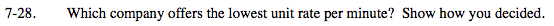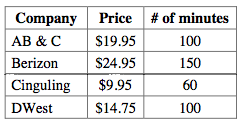### Home > MC2 > Chapter Ch7 > Lesson 7.1.3 > Problem7-28

7-28.For each company, find the price for one minute by dividing the cost by the number of minutes.

${\frac{19.95\ \text{dollars}}{100\ \text{minutes}}=0.1995 \frac{\text{dollars}}{\text{minute}}}$